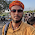## Sunday, 5 April 2015

### Factorial of a Large Number

Idea : - we are going to find factorial of a large number number.Factorial of large number

• store num in array such that each element of array is a single digit.
• Decrease num by 1 and multiply num with each element of array and then for each element we store them as the rightmost digit remains at it's location while the remaining part is added to the next element and the last element is again stored as single digit and the remaining is stored on next till remaining part becomes 0.

### C-Program to find factorial of large number :

1. //C Program to find factorial of very large number
2. #include<stdio.h>
3. #include<stdlib.h>
4. void factorial(int num)
5. {
6.     int temp,i,k=0,a={0};
7.      a=num--;
8.  while(num){
9.     for(i=0;i<=k;i++)
10. /* multiply each element of array with num */
11.     a[i]*=num;
12. /* make each element of array as a single digit */
13.     for(i=0;i<=k;i++)
14.      {
15.         temp=a[i]/10;
16.         a[i]=a[i]%10;
17.         a[i+1]=temp+a[i+1];
18.   /* if i=k then we have reached the last element of array so */
19.         if(i==k)
20.         {
21.          temp=a[k+1];
22. /* we again split the a[k] into single digit and */
23.     while(temp>0)
24.     {
25.       k++;
26.  /* remaining part is stored in next location  */
27.       a[k]=temp%10;
28.       temp=temp/10;
29.     }
30.  /* now set i=k as number of element in array is i now */
31.     i=k;
32.     }
33.   }
34.   num--;
35.  }
36.  for(i=k;i>=0;i--)
37.  printf("%d",a[i]);
38. }
39. main()
40. {
41.  int num;
42.     printf("Enter the number : ");
43.     scanf("%d",&num);
44.     factorial(num);
45.     printf("\n");
46.     system("pause");
47. }

1.Thanks bro for this

1.jo bhi hai accha h :P

2.Very helpful tutorial.I have interest in programming language but most of the time i did not get the tutorials or example.But this blog giving the correct information.I am not an expert in wring so i took the guidelines from the custom essay service.

THANKS FOR UR GREAT COMMENT

Blogger Widgets